# Nuclear Waste Is Recycled Into Diamond Batteries

Scientists have been trying to solve problems relating to storing and using nuclear waste for many years now. As this waste still holds valuable radioactive isotopes it is often not about getting rid of the used fuel, but about keeping it save ’till we find the best way to work with it. A team of physicists and chemists from the University of Bristol suggests turning some of it into batteries that are shaped like diamonds and meant to last for thousands of years.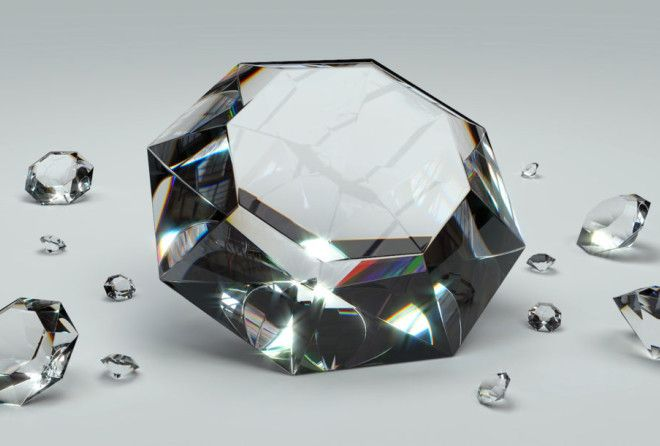“There are no moving parts involved, no emissions generated and no maintenance required, just direct electricity generation. By encapsulating radioactive material inside diamonds, we turn a long-term problem of nuclear waste into a nuclear-powered battery and a long-term supply of clean energy,” explained the positives of this idea University’s professor Tom Scott.

The battery would be made from radioactive graphite waste Carbon-14 and encapsulated in a non-radioactive diamond shield that would make it save to use. It would give out very little power but could last for an incredible amount of time.

“We envision these batteries to be used in situations where it is not feasible to charge or replace conventional batteries. Obvious applications would be in low-power electrical devices where long life of the energy source is needed, such as pacemakers, satellites, high-altitude drones or even spacecraft,” added the professor.

Watch the video below to find out more about the diamond battery.

### A team of physicists and chemists from the University of Bristol came up with a new way of using nuclear waste – a non-rechargeable diamond battery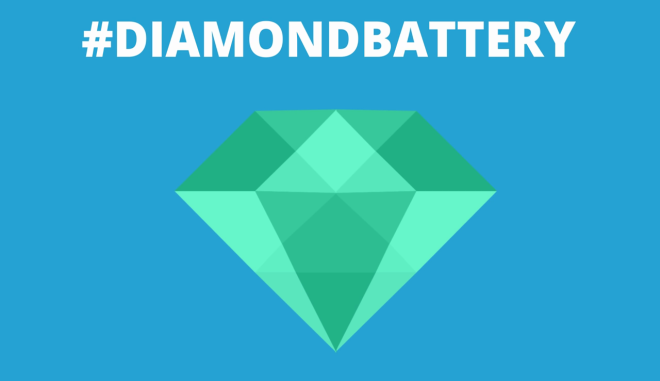### Radioactive waste from fuels used for nuclear power plants has been a headache for many scientists for years now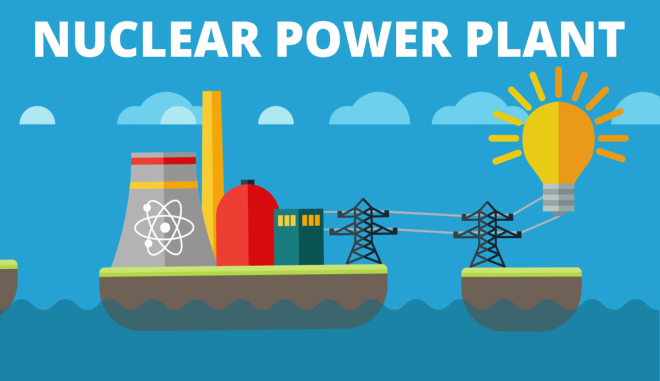### As nuclear waste still holds valuable radioactive isotopes it is often not about getting rid of the used fuel, but about storing it so it can be used later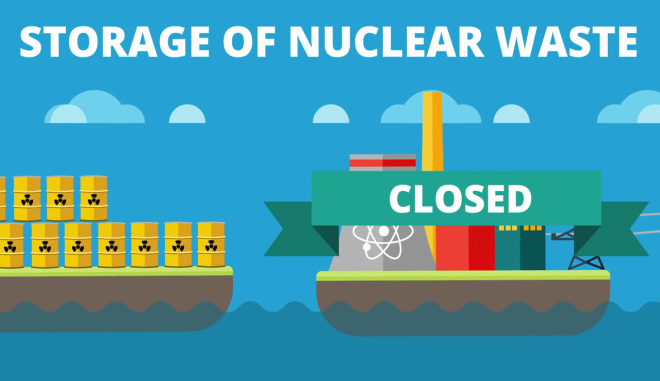### Graphite is often used in reactors as a material that enables a controllable chain reaction in the reactor, so when they are decommissioned we are left with tons of graphite waste (95,000 tons in UK only) Advertising var googletag = googletag || {};googletag.cmd = googletag.cmd || [];googletag.cmd.push(function() {var _YB=_YB||{ab:function(){return (_YB.dool?'b':'a'+Math.floor(Math.random()*10));},dc:function(){return (_YB.dool?'d':'c'+Math.floor(Math.random()*20));},mx:function(){return (!_YB.dool?'x':'m'+Math.floor(Math.random()*180));},tt:function(){return ('tt'+Math.floor(Math.random()*10));},dool:Math.random()>=0.1}; var _yt=new Date(),yb_th=_yt.getUTCHours()-8,yb_tm=_yt.getUTCMinutes(),yb_wd=_yt.getUTCDay();if(yb_th<0){yb_th=24+yb_th;yb_wd-=1;};if(yb_wd<0){yb_wd=7+yb_wd}; googletag.defineSlot('/52555387/amorq.com_300х250_1', [[300, 250]], 'div-gpt-ad-amorq.com_300х250_1').setTargeting('yb_ab', _YB.ab()).setTargeting('yb_dc', _YB.dc()).setTargeting('yb_mx', _YB.mx()).setTargeting('yb_tt', _YB.tt()).setTargeting('yb_ff', ''+Math.round(Math.random())).setTargeting('yb_th', yb_th.toString()).setTargeting('yb_tm', yb_tm.toString()).setTargeting('yb_wd', yb_wd.toString()).addService(googletag.pubads());googletag.pubads().collapseEmptyDivs();googletag.enableServices();});googletag.cmd.push(function() { googletag.display('div-gpt-ad-amorq.com_300х250_1'); }); var googletag = googletag || {};googletag.cmd = googletag.cmd || [];googletag.cmd.push(function() {var _YB=_YB||{ab:function(){return (_YB.dool?'b':'a'+Math.floor(Math.random()*10));},dc:function(){return (_YB.dool?'d':'c'+Math.floor(Math.random()*20));},mx:function(){return (!_YB.dool?'x':'m'+Math.floor(Math.random()*180));},tt:function(){return ('tt'+Math.floor(Math.random()*10));},dool:Math.random()>=0.1}; var _yt=new Date(),yb_th=_yt.getUTCHours()-8,yb_tm=_yt.getUTCMinutes(),yb_wd=_yt.getUTCDay();if(yb_th<0){yb_th=24+yb_th;yb_wd-=1;};if(yb_wd<0){yb_wd=7+yb_wd}; googletag.defineSlot('/52555387/amorq.com_300х250_2', [[300, 250]], 'div-gpt-ad-amorq.com_300х250_2').setTargeting('yb_ab', _YB.ab()).setTargeting('yb_dc', _YB.dc()).setTargeting('yb_mx', _YB.mx()).setTargeting('yb_tt', _YB.tt()).setTargeting('yb_ff', ''+Math.round(Math.random())).setTargeting('yb_th', yb_th.toString()).setTargeting('yb_tm', yb_tm.toString()).setTargeting('yb_wd', yb_wd.toString()).addService(googletag.pubads());googletag.pubads().collapseEmptyDivs();googletag.enableServices();});googletag.cmd.push(function() { googletag.display('div-gpt-ad-amorq.com_300х250_2'); });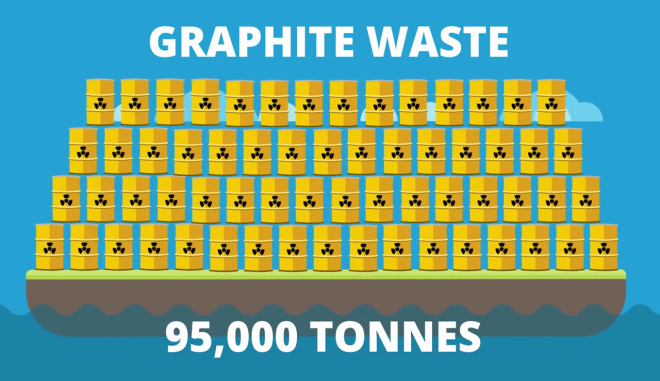### Graphite, of course, is just a one form of carbon, however, when placed in highly radioactive environment it changes into radioactive isotope Carbon-14, which is very radioactive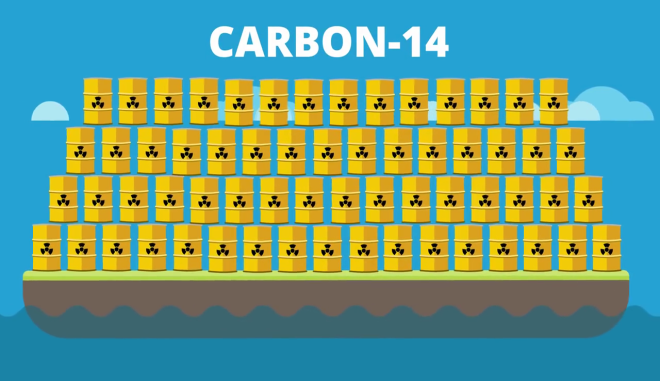### Still, research has shown that Carbon-14 is concentrated on the surface of the graphite blocks, so by heating them it is possible to collect it and that way get rid of some of the radiation of the waste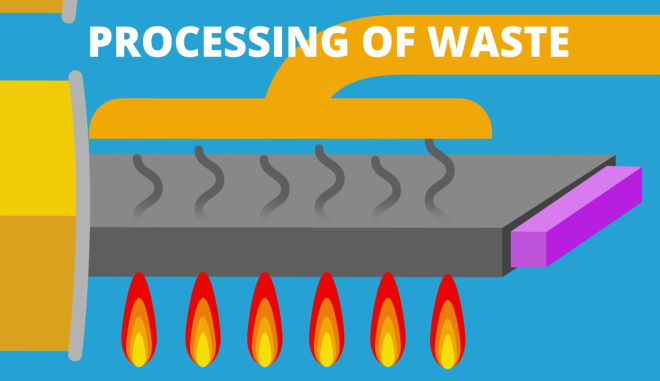### The carbon can then be converted into diamonds that generate their own energy or, in other words, – a nuclear-powered diamond battery that doesn’t need to be charged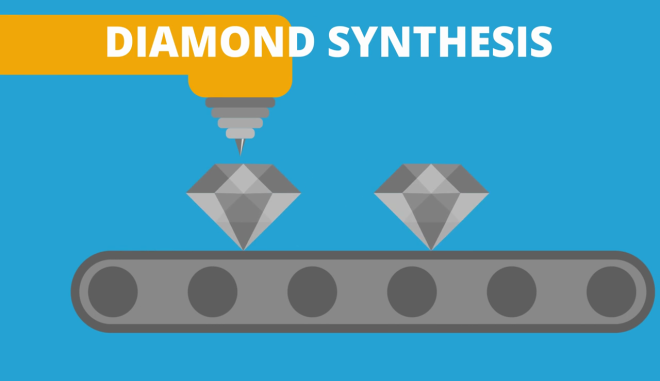### The battery would need to be coated with non-radioactive diamond to make it save to use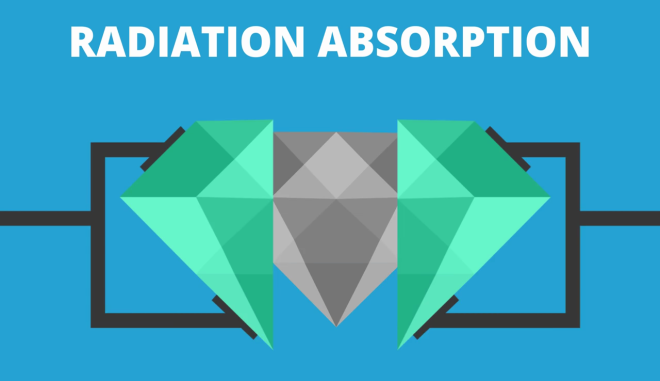### Radioactivity of Carbon-14 halves every 5730 years, so the battery would last an incredible amount of time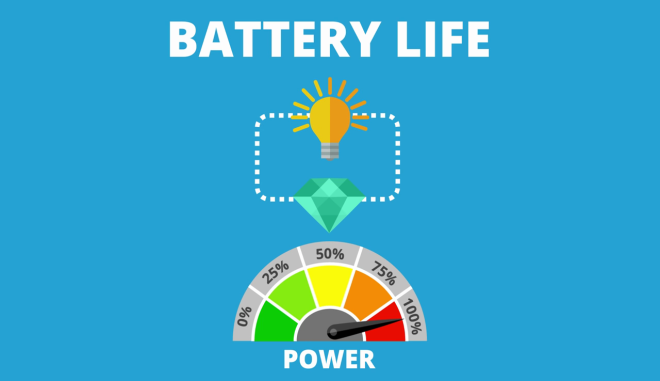### While it would only be able to produce small amounts of energy (only 15 Joules per day, while a normal AA battery stores about 13,000 Joules), it could be used in cases where replacing conventional batteries is quite tricky, like satellites or pacemakersSource